# Flutter Canvas API: Getting Started

Learn how to use the Flutter Canvas API to draw shapes and create custom widgets.

#### Version

• Dart 2.12, Flutter 2.2, Android Studio 4.2

Flutter offers a cross-platform UI toolkit that’s both “expressive and flexible”. But even with this rich and flexible toolkit, there isn’t always an out-of-the-box widget for every nifty design your UI/UX team might dream up. So the Flutter team came up with a solution; they created a canvas and said: “Draw anything you like.” This article will be an entry-level answer to the question: “What is a Flutter canvas, and how can I draw with it?”

In summary, you’ll learn how to:

• Draw basic shapes like polygons, ovals, circles, arcs and curves.
• Compose the basic shapes to draw more complex shapes.
• Animate the complex shapes.

## Drawing 2-D Images on the Screen

Like an artist’s canvas is a physical surface to draw on, a `Canvas` in Flutter is a virtual surface for drawing. But unlike a regular art canvas, you can’t paint on the Flutter canvas with physical brushes.

Flutter `Canvas` uses a two-point (x and y) coordinate system to determine the position of a point on the screen. This coordinate system is a Cartesian coordinate system in two-dimensional space. Although the x-axis starts from the left of the canvas and increases horizontally to the right, the y-axis begins at the top and increases as you move down the canvas. The first pixel at the top-left of the canvas is (0, 0). This position is the origin of both the x-axis and y-axis. Positive numbers move the virtual “brush” right on the x-axis and down on the y-axis. The opposite happens for negative numbers.

To draw, say, a triangle, you pass instructions to the `Canvas` API to start from the coordinate of A, move to C, then move again to B and finally close the gap at A.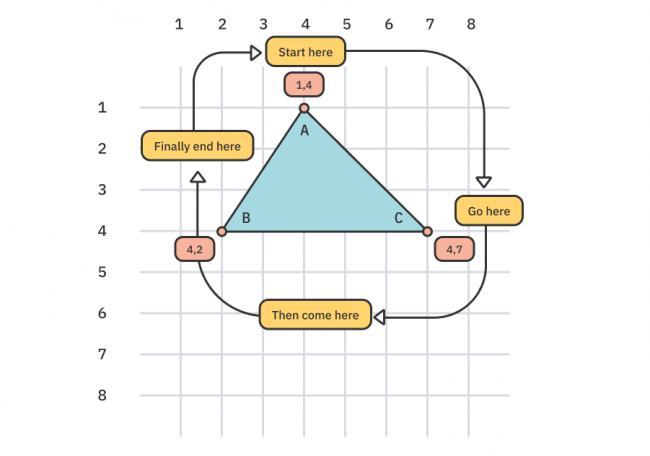## Getting Started

Download the starter project by clicking Download Materials at the top or bottom of this tutorial.

Open the starter folder with the latest version of Android Studio or Visual Studio Code. Next, either run `flutter pub get` in the terminal or open pubspec.yaml and click the Pub get tab that appears in your IDE. Once complete, open lib/main.dart and run the project to see this on your target emulator or device: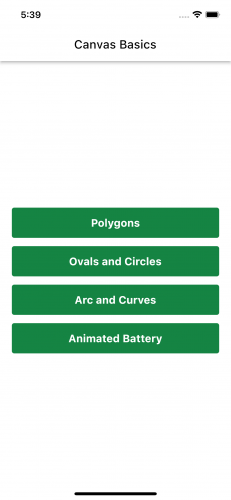Now that you’ve got the project running, it’s time to dive into the canvas API.

## Overview of the CustomPaint and Canvas API

Flutter’s UI rendering stack comprises the Dart and C++ layers. The Material and Cupertino widgets comply with the Google Material and iOS design languages at the Dart layer. This high-level set of widgets depends on the Widgets layer, which depends on the Dart rendering engine. This rendering engine is built from the Animation, Gestures and Painting API, which all lie on the Foundation layer. These layers are written in Dart and powered by a much-lower-level rendering engine written in C++ that uses the high-performance C++ 2D rendering engine, Skia. This lower-level rendering layer exists at the same level as other layers as Platform Channel, Text Layout, System Calls, etc. For more information, see Flutter’s Architectural layers documentation.

`CustomPainter` is an abstract class in the Dart rendering layer. It provides you with a `Canvas`. This `Canvas` and most of the other APIs you’ll use to draw come from the Dart painting layer.

You’ll extend this class to draw your custom shape. A minimal implementation looks something like this:

```class MyFancyPainter extends CustomPainter {

@override
void paint(Canvas canvas, Size size) {

}

@override
bool shouldRepaint(covariant CustomPainter oldDelegate) => false;
}
```

In `paint`, The `size` parameter contains the width and height of the `Canvas` in logical pixels. You’ll use this to determine the size of the shapes to draw. If you draw outside this `size`, the shapes will cut off or, worse still, won’t be visible on the screen. `shouldRepaint()` is called when a new instance of the custom painter is created. This helps the Flutter engine decide whether to call `paint()` again.

To display the shapes drawn in a `CustomPainter`, you need a `CustomPaint` widget. `CustomPaint` is just like a normal widget. It accepts an instance of a subclassed `CustomPainter` in its `painter` and `foregroundPainter` parameters. Next, is accepts a `child` parameter which can be any widget. In some ways, `CustomPaint` behaves like a `Stack` widget. The value of `painter` renders first, then `child`, and `foregroundPainter` renders on top. All three parameters are optional.

## Drawing Basic Shapes

You’ll continue exploring the `Canvas` API by writing instructions to draw basic shapes like polygons, ovals, circles, arcs and curves.

### Drawing Polygons

Before drawing on the canvas, you need to decide the color and size of the drawing pen. An instance of `Paint` defines the pen. Add the following lines of code to `PolygonPainter`‘s `paint()` in the file lib/polygon_painter.dart.

```final paint = Paint()
..strokeWidth = 5
..color = Colors.indigoAccent
..style = PaintingStyle.stroke;
```

Here’s a breakdown of the fields you just set:

• strokeWidth: The width, in pixels, of the drawing pen.
• color: The color of the drawing pen.
• style: Whether the shape should be filled in or just drawn as an outline.

There are other properties for `Paint`, but those are the ones you’ll need for this tutorial.

Next, you’ll learn how to draw a triangle.

#### Drawing a Triangle

Now, you’ll draw a triangle on the canvas. Run the project and tap the Polygons button to see this: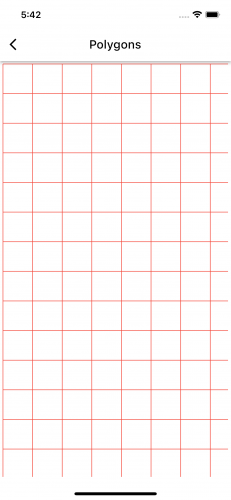The screen has a grid as a visual aid. The grid divides the area into boxes like graph paper. Each box is 50px by 50px.

Note: The grid display uses the same graphics primitive routines you’ll learn in this tutorial. After completing the tutorial, review the grid code in `GridPainter` and `GridWidget` in lib/grid to see how to draw the grid.

You’ll need to place your pen somewhere on the `Canvas` to start drawing. Then, you’ll declare a `Path` object. Afterward, you’ll move the drawing pen to three boxes from the left and zero boxes from the top. And because each box is 50px by 50px, that’s 150px from the left and 0px from the top. Add the following code below the `paint` declaration in the `paint()` of the `PolygonPainter`.

```final triangle = Path();
triangle.moveTo(150, 0);
```

Hot-reload the app, and you’ll notice nothing changed. This is because you have to tell the `Canvas` object to draw the `triangle` path. Add the following below the triangle `path` you just declared:

```canvas.drawPath(triangle, paint);
```

Notice that you also passed `paint into drawPath. The Flutter engine will use the paint object to draw the actual triangle.`

Hot-reload the app again, and you'll still notice nothing changed.What's the reason this time? Technically, you haven't drawn anything yet. You only moved your pen to a point on the `Canvas`.

Now, you need three lines to draw a triangle. To draw the first line, you'll need to move your pen from the current point to another. Add this piece of code below the call to `moveTo()`:

```triangle.relativeLineTo(100, 100);
```

This instruction draws a line from where you placed your pen earlier to two boxes to the right and two boxes below.

Hot-reload the app to see this: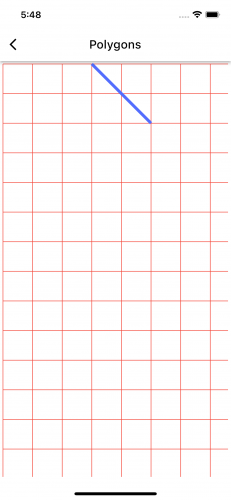To draw the second line of the triangle, add this method call after the previous `relativeLineTo` statement:

```triangle.relativeLineTo(-150, 0);
```

This instruction draws a line from where you stopped earlier to three boxes to the left while remaining at the same vertical position. Notice how left move commands use negative values?

Hot-reload the app again, and you'll see this: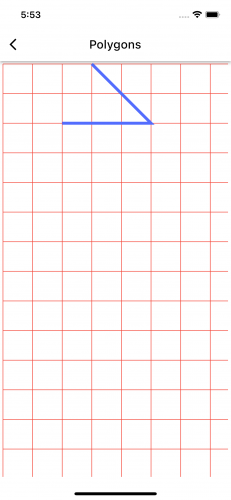You need another line to complete the triangle. You could either draw this line manually like you've been doing earlier or use `Path` `close()`. The latter automatically draws a line from the current position of the pen to where you initially moved your pen.

Now, write this below the other triangle path method calls:

```triangle.close();
```

Your PolygonPainter paint method should now look like this:

```  void paint(Canvas canvas, Size size) {
final paint = Paint()
..strokeWidth = 5
..color = Colors.indigoAccent
..style = PaintingStyle.stroke;

final triangle = Path();
triangle.moveTo(150, 0);
triangle.relativeLineTo(100, 100);
triangle.relativeLineTo(-150, 0);
triangle.close();

canvas.drawPath(triangle, paint);
}
```

Hot-reload the app and you'll get this: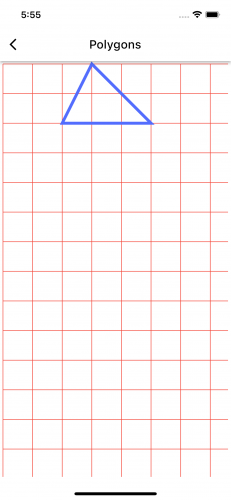Now you'll learn how to draw a square.

#### Drawing a Square

Like drawing with a physical canvas and brushes, there are several ways to draw a shape. A good example is a four-sided polygon like a square or rectangle. You could draw the individual lines from one point to another until you complete the shape or use the `Rect` object.

1. The `Path` Object Method:
```  final square1 = Path();
// 1
square1.moveTo(50, 150);
// 2
square1.relativeLineTo(100, 0);
// 3
square1.relativeLineTo(0, 100);
// 4
square1.relativeLineTo(-100, 0);
// 5
square1.close();
```

Here's a breakdown of that code:

1. Start at one box from the left and three boxes from the top.
2. From there, draw a line to two boxes to the right while remaining at the same y point (i.e., a horizontal line).
3. From there, draw a line to two boxes downwards while remaining at the same x point (i.e., a vertical line).
4. From there, draw a horizontal line to two boxes to the left.
5. Join the current position to the starting position.
2. The `Rect` Object Method:
`const square2 = Rect.fromLTWH(200, 150, 100, 100);`

This draws a 100px by 100px shape from four boxes from the left and three boxes from the top.

Both methods will draw the same shape but at different positions. Add the code from both the rectangle draw methods above inside the `paint()` of `PolygonPainter` after the `canvas.drawPath(triangle, paint)` statement. Then, add the following two statements to draw each of the rectangles:

```canvas.drawPath(square1, paint);
canvas.drawRect(square2, paint);
```

Hot-reload the project to see this: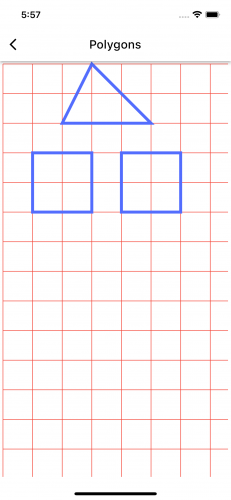Can you guess which method drew which square?

Now that you've mastered squares, you can move on to hexagons.

#### Drawing a Hexagon

To draw a hexagon — a polygon with six sides — use the `Path` object like you did in the first method above to draw a square. Add the following statements below the other drawing instructions in the `paint()` of `PolygonPainter`:

```final hexagon = Path()
// 1
..moveTo(175, 300)
// 2
..relativeLineTo(75, 50)
// 3
..relativeLineTo(0, 75)
// 4
..relativeLineTo(-75, 50)
// 5
..relativeLineTo(-75, -50)
// 6
..relativeLineTo(0, -75)
// 7
..close();
canvas.drawPath(hexagon, paint);
```

Here's what that code is doing:

1. Start at 175px from the left and 300px from the top.
2. From there, draw a line to a point at 75px on the x-axis and 50px on the y-axis.
3. From there, draw a 75px vertical line to downwards.
4. From there, draw a line to a point -75px on the x-axis and 50px on the y-axis.
5. From there, draw a line to a point -75px on the x-axis and -50px on the y-axis. Negative values move up on the y-axis.
6. From there, draw a 75px vertical line upward.
7. Join the current position to the starting position.

Hot-reload, and you'll see this: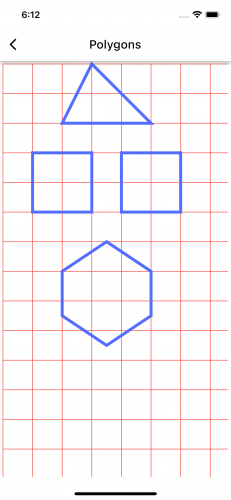Hexagons are great, but wouldn't it be cool if you could draw more complex objects? Good news! You'll learn how to draw more complex objects next.

#### Drawing a Cross - a More Complex Polygon

You can also draw even more complex shapes like a Greek Cross with many joints by moving from one point to another. Add the statements below inside `paint()` of `PolygonPainter`:

```final cross = Path()
..moveTo(150, 500)
..relativeLineTo(50, 0)
..relativeLineTo(0, 50)
..relativeLineTo(50, 0)
..relativeLineTo(0, 50)
..relativeLineTo(-50, 0)
..relativeLineTo(0, 50)
..relativeLineTo(-50, 0)
..relativeLineTo(0, -50)
..relativeLineTo(-50, 0)
..relativeLineTo(0, -50)
..relativeLineTo(50, 0)
..close();
canvas.drawPath(cross, paint);
```

Hot-reload to see this: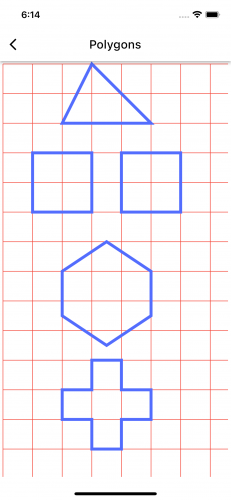That's just the basics of drawing custom shapes: You draw lines from one position to another. But what if you want solid shapes and not just lines? That, too, is simple! Change the `style` property of the `paint` instance from `PaintingStyle.stroke` to `PaintingStyle.fill`. Hot-reload to see solid shapes like these: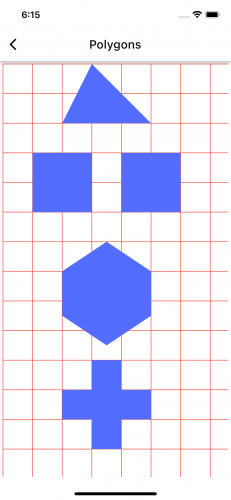Next up, you'll learn about drawing circles.

### Drawing Circles

Start off by opening the lib/ovals_painter.dart file. To draw your oval you'll need another instance of `paint`, so add the following below in `paint()`:

```final paint = Paint()
..strokeWidth = 4
..color = Colors.blueAccent
..style = PaintingStyle.stroke;
```

Unlike the straight lines that make up polygons, drawing circles and ovals doesn't require moving from point to point on the `Canvas`. One of the easiest ways of drawing a perfect circle is to define the center point of the circle and give it a radius. Add the following below the `paint` declaration:

```const circleRadius = 75.0;
const circleCenter = Offset(200, 150);
```

The center of the circle is at 200px from the left and 150px from the top. The radius of the circle is 75px.

Run the app and tap the Ovals and Circles button to see this displayed in the app: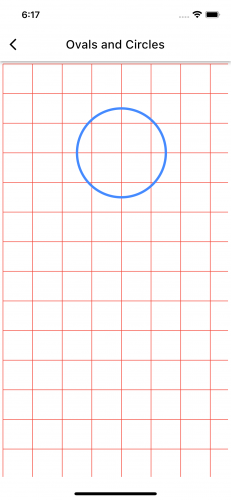Now onto ovals!

## Drawing Ovals

Ovals are just circles but with unequal width and height.

Add the following oval drawing statements below the code for drawing the circle in `OvalPainter`:

```const ovalCenter = Offset(200, 275);
final oval = Rect.fromCenter(center: ovalCenter, width: 250, height: 100);
canvas.drawOval(oval, paint);
```

The center of the oval will be at 200px from the left and 275px from the top. It'll be 250px wide and 100px tall.

Run the project to see this: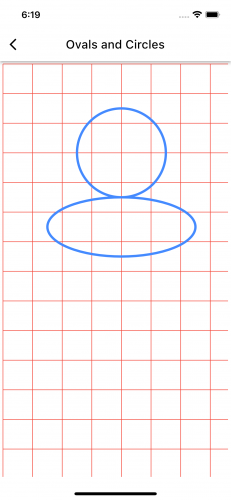You have drawn an avatar by combining a circle and an oval! You can also combine circles to draw concentric circles. To achieve this, you can iteratively reduce the radius of the circle.

Add the following concentric circle code below the instructions for drawing the oval:

```var concentricCircleRadius = 100.0;
const center = Offset(200, 500);
while (concentricCircleRadius > 0) {
}
```

Hot-reload and you'll see this: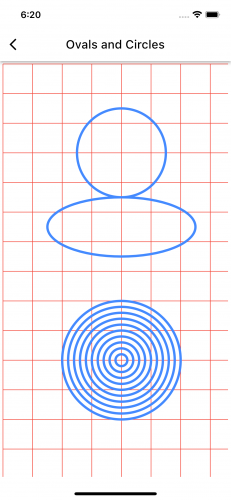You'll do more shape composition in later sections.

### Drawing Arcs and Curves

The `Canvas` API supports four types of curves: arc, quadratic Bézier, cubic Bézier and conic Bézier. In this section, you'll learn about arcs and quadratic and cubic Bézier curves.

#### Arc

An arc is a section of a circle. Imagine a circular pizza. After you've eaten a slice from it, what's left is an arc. The slice you ate is also an arc. The angle made by the slice of pizza when the slice is equal to the radius of the whole pizza is called the radian.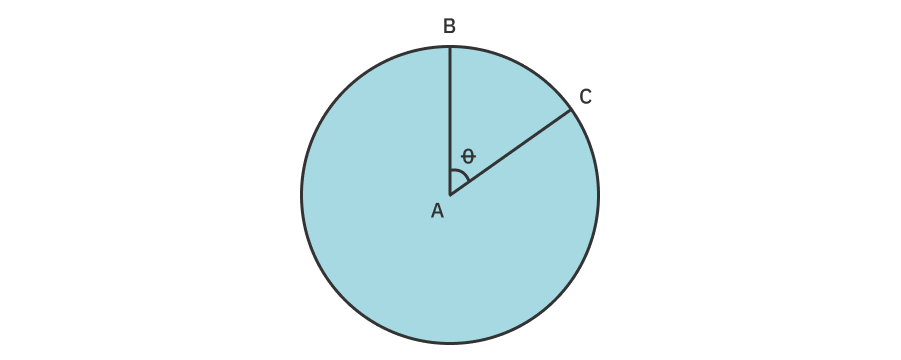In the diagram above, the angle of θ is defined as one radian if the arc length made by BC equals the circle's radius.

You need a bounding box, a start angle and a sweep angle to draw an arc. Both start and sweep angles are in radians. Because many people like to think of angles in units of degrees, the utility function `degreesToRadians()` has been provided in the `ArcsCurvesPainter` class to convert degrees to radians.

The start angle begins at the x-axis on the circle, as shown by the red line shown below. You draw positive angles in the clockwise direction. The end position of the arc is the sum of start and sweep angles.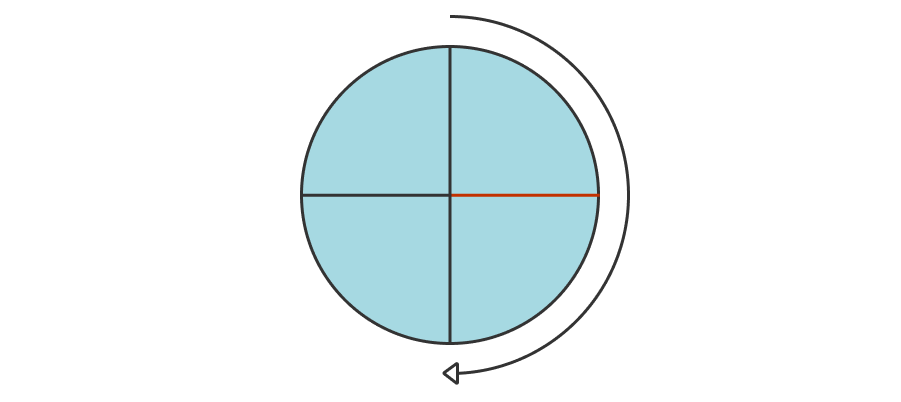Add the following lines of code to the `paint()` of `ArcsCurvesPainter` in arcs_curves_painter.dart:

```const arcCenter = Offset(200, 80);
final arcRect = Rect.fromCircle(center: arcCenter, radius: 75);
final startAngle = degreesToRadians(10);
final sweepAngle = degreesToRadians(90);
canvas.drawArc(arcRect, startAngle, sweepAngle, true, curvesPaint);
```

Run the project and tap the Arc and Curves button. You'll see an arc drawn in the clockwise direction: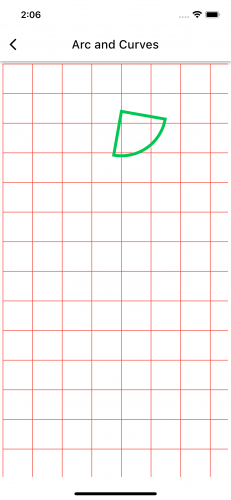To draw the arc counterclockwise, change the sweep angle to a negative number:

```final sweepAngle = degreesToRadians(-90);
```

Hot-reload, and you'll see this: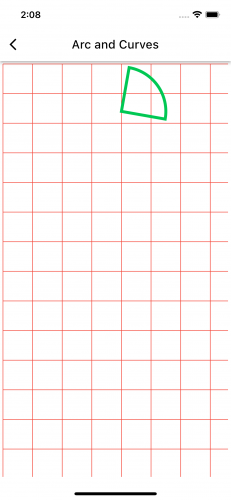The third parameter of `canvas.drawArc()` determines how to close the arc. Passing `true`, as you did, draws the arc like a slice of pizza. Otherwise, it draws just the curved line segment.

Change the drawing instruction of the arc to this:

```canvas.drawArc(arcRect, startAngle, sweepAngle, false, curvesPaint);
```

Hot-reload to see this: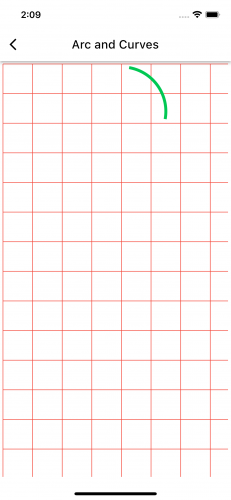Play around with the parameters to better grasp how `canvas.drawArc()` behaves.

Onwards to Bézier curves!

#### Quadratic Bézier Curves

Quadratic Bézier curves are sub-path segments that curve from the current point to an endpoint using a control point. The control point defines the slope at the beginning and the end of the curve.

Here's an example. The curve starts at point A (50, 300) and ends at B (350, 300). Point C is the control point at (150, 200). The plotted Bézier curve is the brown line.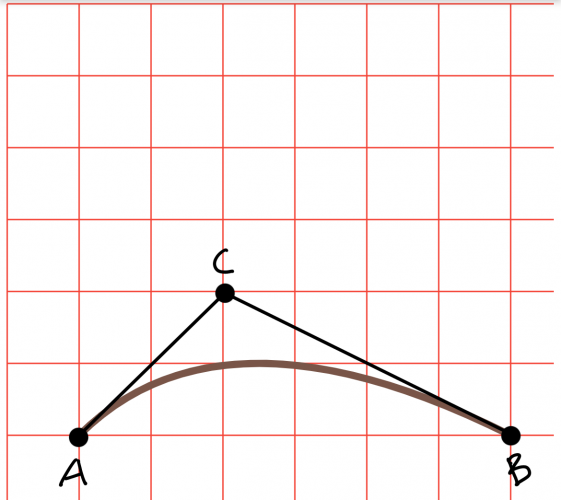And here's the Dart code for this curve:

```final qCurve1 = Path()
..moveTo(50, 300)
..relativeQuadraticBezierTo(100, -100, 300, 0);
canvas.drawPath(qCurve1, curvesPaint);
```

Look at another example:

The curve starts at (50, 50) and ends at (350, 150), and the control point is at (200, 350). As illustrated by the image below, A is the starting point, C is the control point and B is the end of the line. The plotted Bézier curve is the brown line.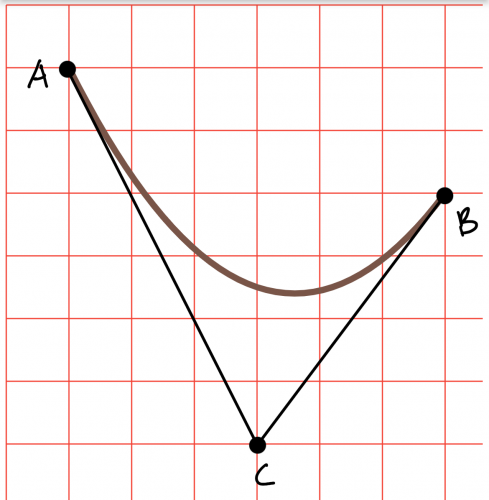```final qCurve2 = Path()
..moveTo(50, 50)
..relativeQuadraticBezierTo(150, 300, 300, 100);
canvas.drawPath(qCurve2, curvesPaint);
```

#### Cubic Bézier Curves

Although Quadratic Bézier uses one control point, Cubic Bézier uses two control points, giving more control over the beginning and end slope of the curve. Here's a simple example: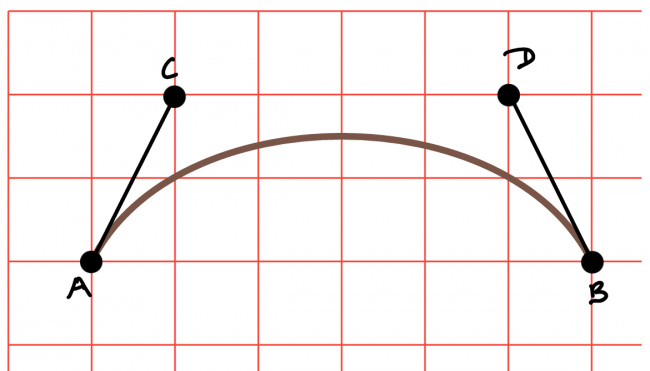The curve starts at (50, 150) and ends at (350, 150). The curve begins with a slope defined by the line AC and ends with the slope defined by the line DB.

```final cCurve1 = Path()
..moveTo(50, 150)
..relativeCubicTo(50, -100, 250, -100, 300, 0);
canvas.drawPath(cCurve1, curvesPaint);
```

Here's another example. The curve starts at (350, 50) and ends at (200, 300). Like the previous example, the curve begins with a slope defined by the line AC and ends with the slope defined by the line DB.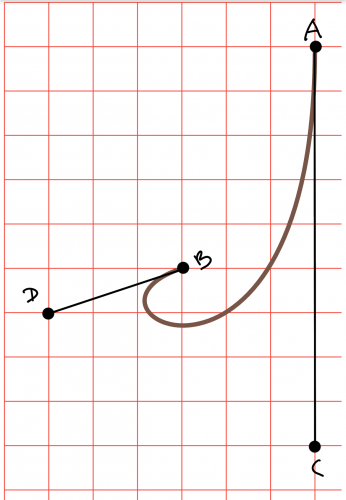```final cCurve2 = Path()
..moveTo(350, 50)
..relativeCubicTo(0, 450, -300, 300, -150, 250);
canvas.drawPath(cCurve2, curvesPaint);
```

To run the Bézier curve examples, add the following lines of code to `paint()` in `ArcsCurvesPainter`.

```// Quadratic Bézier
final qCurve1 = Path()
..moveTo(50, 150)
..relativeQuadraticBezierTo(100, -100, 300, 0);
canvas.drawPath(qCurve1, curvesPaint..color = Colors.deepPurpleAccent);

final qCurve2 = Path()
..moveTo(0, 150)
..relativeQuadraticBezierTo(150, 300, 300, 100);
canvas.drawPath(qCurve2, curvesPaint..color = Colors.blue);

// Cubic Bézier
final cCurve1 = Path()
..moveTo(0, 450)
..relativeCubicTo(50, -100, 250, -100, 300, 0);
canvas.drawPath(cCurve1, curvesPaint..color = Colors.black);

final cCurve2 = Path()
..moveTo(380, 300)
..relativeCubicTo(0, 450, -300, 300, -150, 250);
canvas.drawPath(cCurve2, curvesPaint..color = Colors.pink);
```
Note: Some of the start points have been modified so the curves fit on a single screen.

Hot-reload to see this: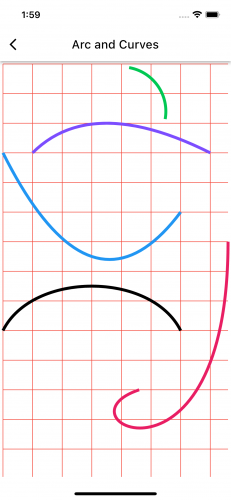## Animating Custom Shapes

Combined with the simplicity of the animation API, you can implement fancy animations with custom shapes. To see how this works, you'll build an animated charging/discharging battery widget in this section.

### Animating a Charging Battery

In this section, you'll draw a charging and discharging battery and drive the charge progress with the animation controller.

Here's what the unanimated state looks like: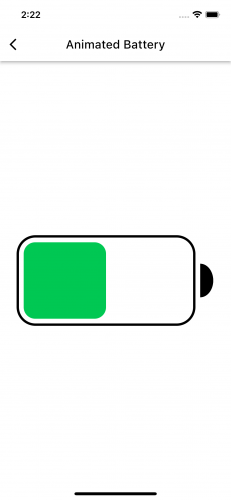The battery consists of the following shapes:

1. A border with rounded corners drawn using `RRect`. An `RRect` is the same as `Rect`, except it supports defining rounded corners.
2. A filled semi-circle drawn with an arc representing the battery pin.
3. The amount of charge drawn with a `RRect`.

Start by opening animated_battery.dart in the battery folder. `AnimatedBattery` has an animation controller configured to animate forever in an endless loop, calling `setState()` when the controller value updates. Inside `build()` is a `CustomPaint` widget. The animation value passed to `BatteryPainter` is used to draw the amount of battery charge.

Run the app and select the Animated Battery button, and you'll see a blank screen.

Open battery_painter.dart in the battery folder. `BatteryPainter` is where you'll draw the battery widget. To move things along, some fields are already declared.

Start by drawing the battery border. Add the following code below `paint()`.

```RRect _borderRRect(Size size) {
// 1
final symmetricalMargin = margin * 2;
// 2
final width = size.width - symmetricalMargin - padding - pinWidth;
// 3
final height = width / 2;
// 4
final top = (size.height / 2) - (height / 2);
// 5
// 6
final bounds = Rect.fromLTWH(margin, top, width, height);
// 7
}
```

Here's a breakdown of that code:

1. The total of the margin on each axis. You're giving the shape a margin of the value `margin` on the top, right and bottom.
2. The width of the border. This is the `Canvas` width minus the horizontal margins, the pin width and the space between the pin and the border.
3. The height of the border. The height is half the width.
4. The top of the border. This value is the y-coordinate of the top edge of the battery that will center the battery on the canvas.
5. The radius of the border. The corner radius is 20 percent of the height.
6. Instantiate the bounds of the border with all the above values. The left offset of the border is the value of the margin. That'll give a space (the value of margin) before drawing the border.
7. Instantiate and return the RRect from the bounds and radius.

Now, add this code inside `paint()`:

```// Battery border
final bdr = _borderRRect(size);
canvas.drawRRect(bdr, borderPaint);
```

Run the code to see this: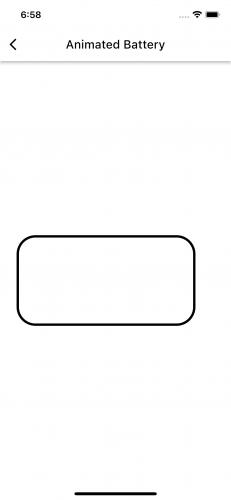Next, you'll draw the battery pin. Add the following code below the declaration of `_borderRRect()`:

```Rect _pinRect(RRect bdr) {
// 1
final center = Offset(bdr.right + padding, bdr.top + (bdr.height / 2.0));
// 2
final height = bdr.height * 0.38;
// 3
final width = pinWidth * 2;
// 4
return Rect.fromCenter(center: center, width: width, height: height);
}
```

Here's what this is doing:

1. The center of the pin. Position the center with the center of the height of the battery border. Add some padding to position the pin away from the right edge of the battery border.
2. The height of the pin, which is 38 percent of the height of the border of the battery.
3. The pin bounding box width. This width is twice the pinWidth. The arc will only occupy half this.
4. Instantiate and return the pin bounds.

Add the following import for the math package.

```import 'dart:math' as math;
```

Now, add the drawing instructions for the battery pin to `paint()` after the battery border:

```// Battery pin
final pinRect = _pinRect(bdr);
canvas.drawArc(pinRect, math.pi / 2, -math.pi, true, pinPaint);
```

This draws a filled arc from 90° to -180°.

Hot-reload, and you'll see this: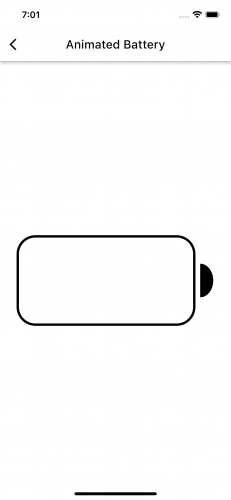The next step is to draw the charge of the battery. In this first iteration, you won't animate the charge. Add this below the declaration of `_pinRect()`:

```RRect _chargeRRect(RRect bdr) {
final left = bdr.left + padding;
final top = bdr.top + padding;
final right = bdr.right - padding;
final bottom = bdr.bottom - padding;
final height = bottom - top;
final width = right - left;
final rect = Rect.fromLTWH(left, top, width, height);
}
```

The statements above compute the bounds of the charge shape using the bounds of the battery border while providing for the padding.

Now, add this below the drawing instructions for the pin in `paint()`:

```// Battery charge progress
final chargeRRect = _chargeRRect(bdr);
canvas.drawRRect(chargeRRect, chargePaint);
```

Run the code to see this: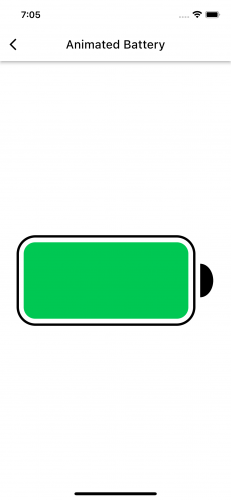The next step is to animate the charge progress, and you won't believe how ridiculously — wait for it! (in the voice of Barney Stinson) — easy this is!

In `_chargeRRect()`, simply replace the width parameter passed to `Rect.fromLTWH()` from `width` to `width * charge`. The line becomes:

```final rect = Rect.fromLTWH(left, top, width * charge, height);
```

Run the project, and you'll see this: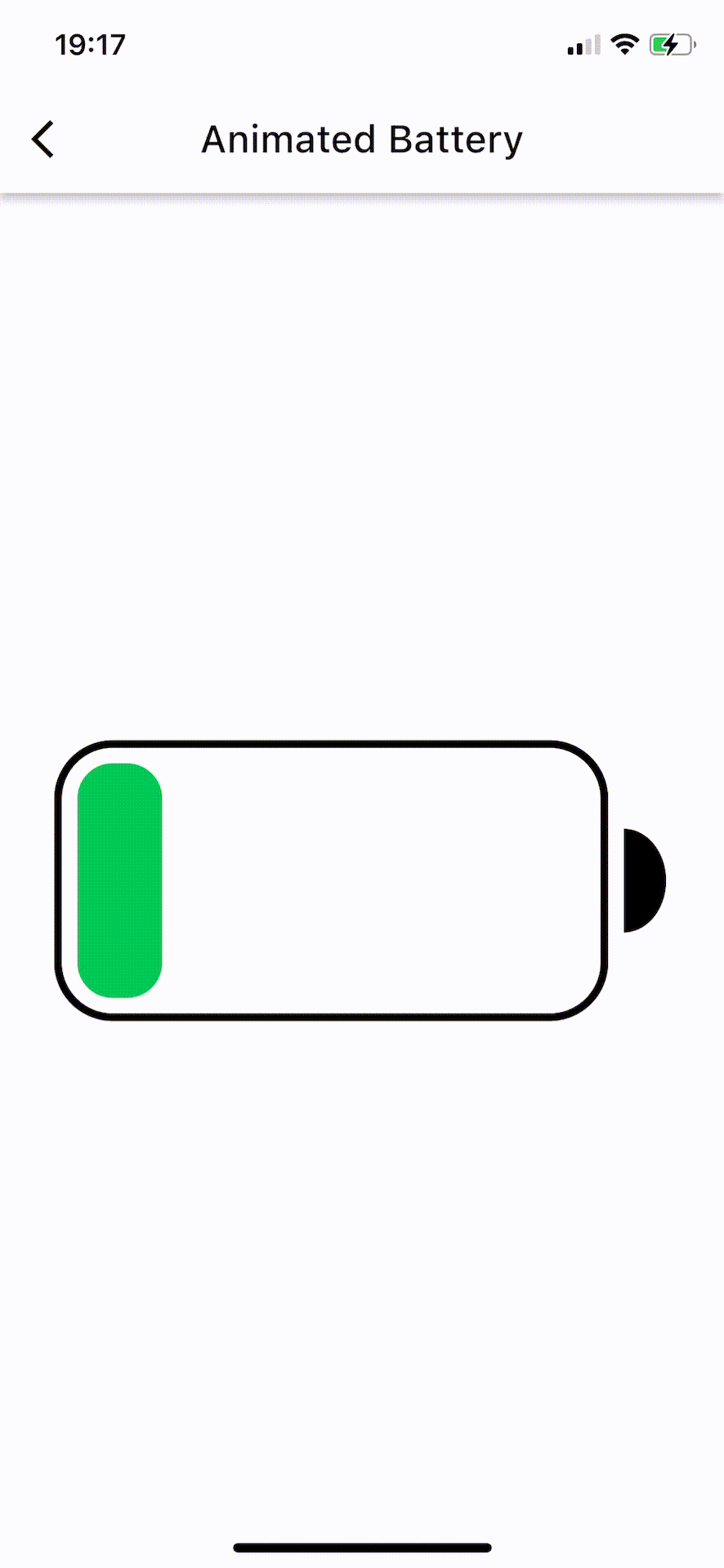In reality, this is not how a battery charges. To fix this, you'll make the animation progress in increments of ` minCharge`.

Write the statement below inside `_chargeRRect()`, just at the top:

```final percent = minCharge * ((charge / minCharge).round());
```

Also, change the width value you just changed from `width * charge` to `width * percent`, so `_chargeRRect()` becomes:

```RRect _chargeRRect(RRect bdr) {
final percent = minCharge * ((charge / minCharge).round());
final left = bdr.left + padding;
final top = bdr.top + padding;
final right = bdr.right - padding;
final bottom = bdr.bottom - padding;
final height = bottom - top;
final width = right - left;
final rect = Rect.fromLTWH(left, top, width * percent, height);
}
```

Run the code to see this: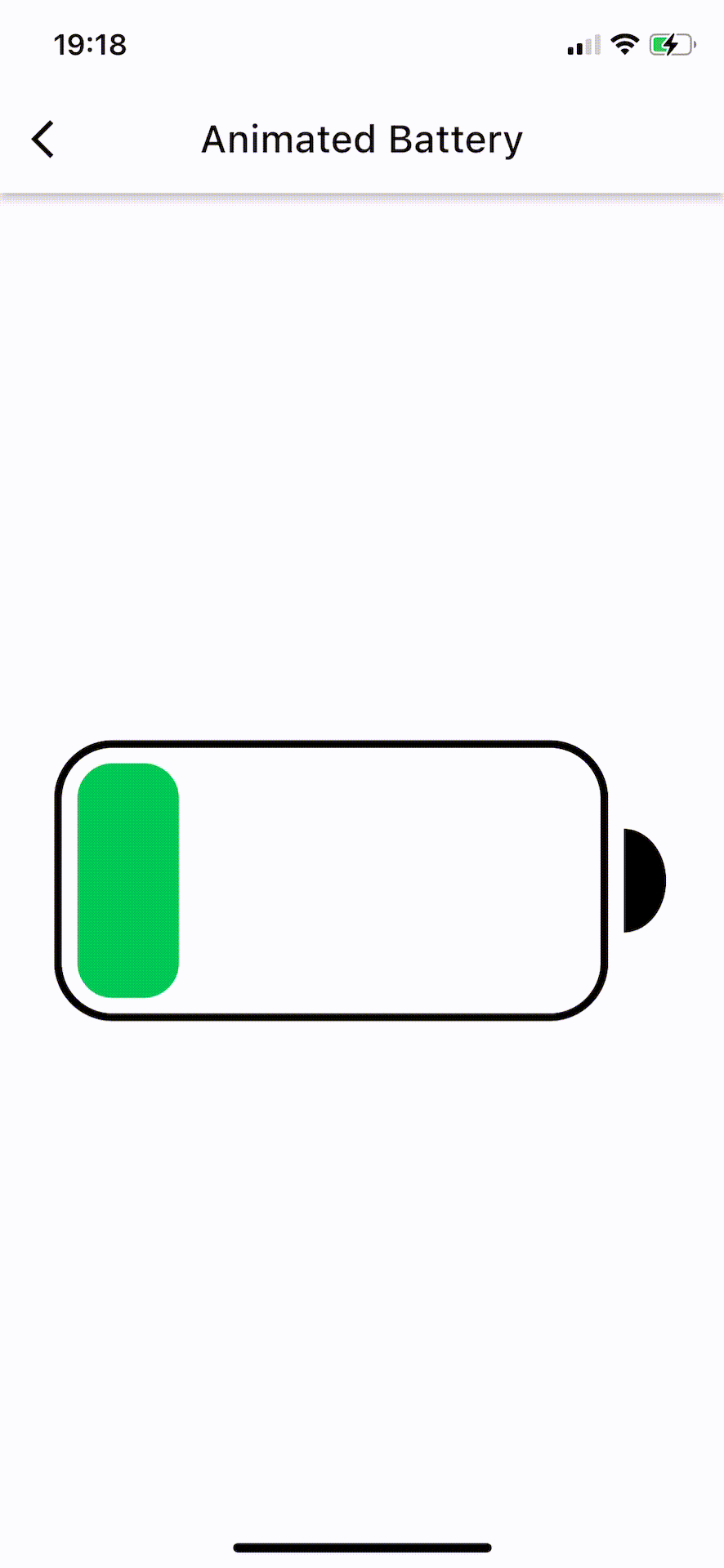That's it! You did such a fantastic job!

## Where to Go From Here?

The completed project contains all the code used in this tutorial. You can find this inside the completed folder in the file you downloaded earlier or by clicking Download Materials at the top or bottom of this tutorial.

This tutorial taught you to draw basic and composite custom shapes with the Flutter `Canvas` API. Additionally, you learned how to tap into the power of the animation API to animate these custom shapes.

To learn more about the Flutter architectural layers, head over to this detailed official documentation by the Flutter team. This Stackoverflow answer by Flutter's former Product Manager Sett Ladd highlights the difference between logical and physical pixels in relationship to Flutter.

I hope you enjoyed this tutorial. If you have any questions or comments, please join the forum discussion below!